Definitions of Square Dance Calls and Concepts

Index -->  Plus  |  A1  |  A2  |  C1  |  C2  |  C3A  |  C3B  |  C4  |  NOL  |
Definitions (Text Only) -->  Plus  |  A1  |  A2  |  C1  |  C2  |  C3A  |  C3B  |  C4  |  NOL  |
 Find call:

Invert The Column ({fraction}) [C2]
(Ed Foote \$B\$H(B Russ McGowan 1974)

From Parallel Columns.

The First n dancers (where n corresponds to the given fraction. E.g., from Parallel Columns of 4, n=1 for 1/4; 2 for 1/2; 3 for 3/4; 4 for full), as a Tandem unit of n, Peel Off to end in Concentric Columns of n as the Trailing dancers move forward (using Circulates) to form a compact formation.

Parallel Columns of 4 \$B\$+\$i(B: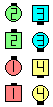Invert The Column\$B\$NA0(B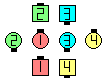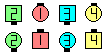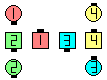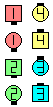1/4\$B\$N8e(B 1/2\$B\$N8e(B 3/4\$B\$N8e(B (\$B=*\$o\$j(B)
Parallel Columns of 3 \$B\$+\$i(B: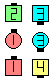Invert The Column\$B\$NA0(B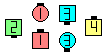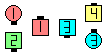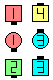1/3\$B\$N8e(B 2/3\$B\$N8e(B (\$B=*\$o\$j(B)

Note: Upon completion of a full Invert The Column, dancers slide together to end in adjacent Columns. Do not slide together if the call is fractionalized (e.g., 3/4 Invert The Column ends in an "H").

Continue To Invert Another fraction [C4]: From one of the intermediate stages of Invert The Column. Do the next fraction parts of Invert The Column.

Variation:Magic Invert The Column (fraction) [C2V].

Magic Invert The Column: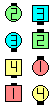Magic Invert The Column\$B\$NA0(B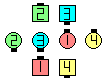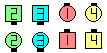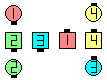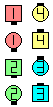1/4\$B\$N8e(B 1/2\$B\$N8e(B 3/4\$B\$N8e(B (\$B=*\$o\$j(B)

Although I have never heard anyone call a (full) Magic Invert The Column, I believe that it should end in normal Columns, not Magic Columns. This is because of the Magic rule that says that after you leave the Magic Column Circulate path, the remainder of the call is finished normally.

\$BCross Invert The Column (fraction) [C2].CALLERLAB definition for Invert the Column 1/4, 1/2, 3/4, FullChoreography for Invert The Column (fraction)Comments? Questions? Suggestions?